# AP Chemistry : Titrations and Indicators

## Example Questions

### Example Question #1 : Titrations And Indicators

At what pH does the equivalence point lie for a strong-acid / strong-base titration?

7

Slightly basic

Slightly acidic

It is impossible to determine

7

Explanation:

The equivalence point for a strong-acid / strong-base titration will be at neutral pH, 7. This is because each equivalent of the acid will neutralize each equivalent of the base, and you will be left with a neutral solution.

### Example Question #1 : Titrations And Indicators

At what pH does the equivalence point lie for a strong-acid / weak-base titration?

It is impossible to determine

7

Slightly acidic

Slightly basic

Slightly acidic

Explanation:

The equivalence point for a strong-acid / weak-base titration will be at a slightly acidic pH. This is because the acid is stronger and dissociates to a greater degree, while the base is not quite as strong, so doesn't dissociate to a large enough extent to neutralize each equivalent of the acid.

### Example Question #1 : Titrations And Indicators

At what pH does the equivalence point lie for a weak acid-strong base titration?

Slightly acidic

7

Slightly basic

It is impossible to determine

Slightly basic

Explanation:

The equivalence point for a weak-acid / strong-base titration will be at a slightly basic pH. This is because the base is stronger and dissociates to a greater degree, while the acid is not quite and strong and doesn't dissociate to a large enough extent to neutralize each equivalent of the base.

### Example Question #1 : Titrations And Indicators

You are given 500 mL of a HCl solution of unknown concentration and you titrate is with 0.0540 M NaOH. It takes 32.1 mL of the NaOH solution to reach your end point. What is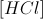of your original solution?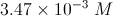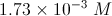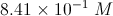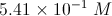Explanation:

First, let us write out the reaction that occurs: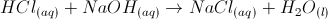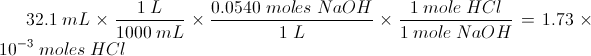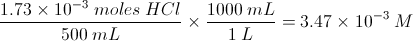### Example Question #6 : Titrations And Indicators

A titration is a drop-by-drop mixing of an acid and a base in order to determine the concentration of an unknown solution, via addition of a solution with known concentration. A titration curve can be graphed showing the relationship between the mixture pH and the amount of known solution added.

What would the titration curve look like for a strong base being titrated with a strong acid?

An increasing sigmoidal curve

A positively sloped line

A decreasing sigmoidal curve

A negatively sloped line

A decreasing sigmoidal curve

Explanation:

There are two things to consider here.

1. Since the solution is originally a strong base, the pH will be originally elevated. As a strong acid is added to the solution, the pH will decrease. As a result, the titration curve will be decreasing as the volume of titrant increases.

2. The titration curve will never be a straight line. Eventually, the strong acid will be much larger in volume than the original base; however, the pH will eventually even out at the pH of the added titrant.

Since we are titrating a strong base with a strong acid, the titration curve will be represented by a decreasing sigmoidal curve.

### Example Question #1 : Titrations And Indicators

Where does the flattest region of a titration curve of the titration of a weak acid with a strong base occur?

At a pH greater than 7

At a pH of 7

At the pKb of the base

At the pKa of the acid

At the pKa of the acid

Explanation:

In this question, titration curve would graph the pH of acid solution versus the amount of base added. Since the base is strong and the acid is weak, we can conclude that the pH will be slightly greater than 7 at the equivalence point. The equivalence point is found in the steepest region of the curve.

The half-equivalence point is the flattest region of the titration curve and is most resistant to changes in pH. This corresponds to the pKa of the acid. Within this region, adding base (changing the x-value) results in very little deviation in the pH (the y-value). This region is also the buffer region for the given acid.

### Example Question #1 : Titrations And Indicators

You have a solution of weak base with unknown concentration. What would be a good acid with which to titrate the weakly alkaline solution, in order to determine its concentration?

Hydrofluoric acid

Citric acid

Stearic acid

Nitric acid

Sodium hydroxide

Nitric acid

Explanation:

When you titrate a weak base, you want to titrate it with a strong acid. Hydrofluoric acid, citric acid, and stearic acid are all weak acids, and sodium hydroxide is a strong base. The best choice it nitric acid, a strong acid.

### Example Question #1 : Titrations And Indicators

You have a 500mL solution of a monoprotic acid with unknown concentration. You titrate it to completion with 36mL of 0.4M NaOH solution. What is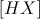?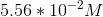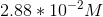There is not enough information to solve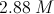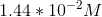Explanation:

If we are working with a monoprotic acid, our chemical equation is: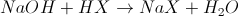Now we will calculate the moles of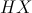in our solution: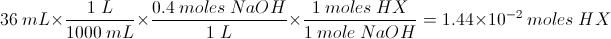Now we will determine the concentration from the amount of moles and the volume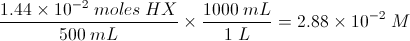### Example Question #51 : Reaction Types

0.458g of an unknown diprotic acid is dissolved in water. It is then titrated with 21.5ml of a 0.500M NaOH solution to reach the second equivalence point. Determine the molecular weight of this unknown acid.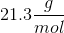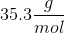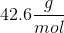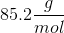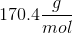Explanation:

To find the molecular weight, we must determine the number of moles that correspond to the 0.458g sample. At the equivalence point, the moles of hydronium ions will equal the moles of hydroxide ions.

We need to use the molarity and volume of the NaOH that was added to find the number of moles of base added. This will tell us the moles of hydroxide ions.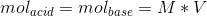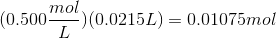Now, since we are working with a diprotic acid, two moles of base would be required for every one mole of the acid. The moles of acid would be: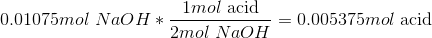Now that we know the moles of acid in the sample, we can use the given sample mass to find the molecular weight.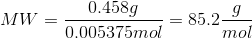### Example Question #81 : Acid Base Reactions

Litmus paper may be used to estimate the pH of a solution colorimetrically. The specific color change observed is dependent on the pH of the solution in which it is submerged.

Based on the following figure, what color would you expect a piece of litmus paper to turn when exposed to a sodium hydroxide solution made by adding 0.004g of anhydrous sodium hydroxide (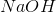) to 1L of water?

Assume the solution's volume is unchanged by the addition of the solid.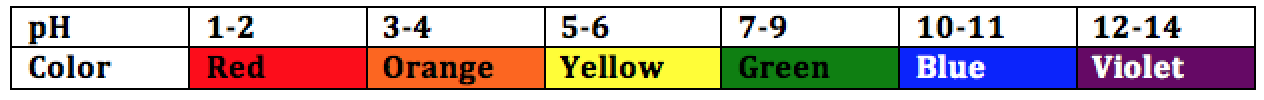Green

Yellow

Orange

Blue

Blue

Explanation:

To determine the predicted color change, the pH of the solution must be determined.

The molar mass ofis approximate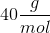.is a strong base and dissociate completely in a 1:1 ratio into its counterions (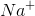and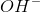).

The concentration ofdetermines the pH of the solution and may be found by calculating the number of moles ofdissolved in solution and dividing by the total volume: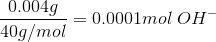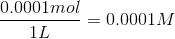Next, we find the pOH of the solution, from which the pH may be easily determined: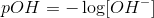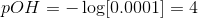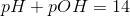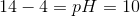According to the given figure, a pH of 10 corresponds to a color change to blue.

### All AP Chemistry Resources